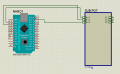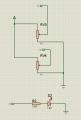Joined Nov 21, 2018
857
Hi,
The circuit below Pin12 flashing when simulate at main sheet, but nothing at sub sheet, why?
Thanks
PWM test:
const int pwmPin = 12; // assigns pin 12 to variable pwm

const int potcyc = A0; // assigns analog pot input A0 to vary the cyc of pwm
const int potfre = A1; // assigns analog pot input A1 to vary the cfrequency of pwm

void setup()  // setup loop
{
pinMode(pwmPin, OUTPUT);
// Don't use pinMode() with analogRead()
}

void loop()
{
float dutyCycleVal = analogRead(potcyc) / 1024.0;
int delayVal = map(analogRead(potfre), 0, 1024, 2000, 0); // delay in

digitalWrite(pwmPin, HIGH);
delayMicroseconds(delayVal * dutyCycleVal); // The ON part of the cycle
digitalWrite(pwmPin, LOW);
delayMicroseconds(delayVal * (1.0 - dutyCycleVal));  // The OFF part of the cycle
}